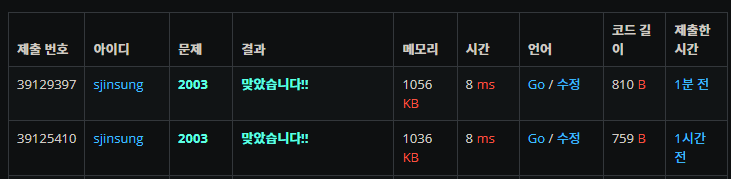# Baekjoon 2003 Sum of numbers in Go

sjinsung·2022년 2월 15일
0

### First

pfunc main() {
var a int
var m int
fmt.Scanf("%d %d\n", &a, &m)
arr := make([]int, a)
result := checker(arr, a, m)
fmt.Print(result)
}

// func ReadN(arr []int, m int) {
// 	for i := 0; i < m; i++ {
// 		fmt.Scan(&arr[i])
// 	}
// 	return
// }

func ReadN(arr []int, i, n int) {
if n == 0 {
return
}
if m, err := fmt.Scan(&arr[i]); m != 1 {
panic(err)
}
}

func checker(arr []int, a, m int) int {
var s int
var e int
var sum int
var result int

for s < a {
if sum > m || e == a {
sum -= arr[s]
s++
} else {
sum += arr[e]
e++
}
if sum == m {
result++
}
}
return result
}
• Scan function didn't wait when I put Enter(\n)
• So I checked and modified like below.
• Enter should be included with Scanf

#### Before

fmt.Scan(&a, &m)

#### After

fmt.Scanf("%d %d\n", &a, &m)

### Second

package main

import (
"fmt"

)

func main() {
var n int //numbers
var m int //target sum

c := make(chan []int)
d := make(chan int)

fmt.Scanf("%d %d\n", &n, &m)
arr := make([]int, n)
go checker(<-c, n, m, d)
fmt.Print(<-d)
}

func ReadN(arr []int, n int, c chan<- []int) {
for i := 0; i < n; i++ {
fmt.Scan(&arr[i])
}
c <- arr
}

func checker(arr []int, n, m int, d chan<- int) {
var start int
var end int
var sum int
var result int

for start < n {
if sum > m || end == n {
sum -= arr[start]
start++
} else {
sum += arr[end]
end++
}
if sum == m {
result++
}
}
d <- result
}

• I added Go routine and changed recursive, but still have problem with the processing time.
package main

import (
"bufio"
"fmt"
"os"
)

func main() {
var n int //numbers
var m int //target sum

fmt.Fscanf(bufin, "%d %d\n", &n, &m)
arr := make([]int, n)
result := checker(arr, n, m)
fmt.Print(bufout, result)
}

for i := 0; i < n; i++ {
fmt.Fscan(bufin, &arr[i])
}
return arr
}

func checker(arr []int, n, m int) int {
var start int
var end int
var sum int
var result int

for start < n {
if sum > m || end == n {
sum -= arr[start]
start++
} else {
sum += arr[end]
end++
}
if sum == m {
result++
}
}
return result
}
• Problem was from Scan and Scanf. It doesn't do any buffering, which makes it very slow if you get a lot of input. So I changed all Scan, Scanf to Fscan, Fscanf. Instead, I used bufio package. However, if you are not satisfied with the speed even with Fscanf(), you should seriously consider using a Scanner. See below code.
• Thank you for comment from Nomadcorder slack!!

#### Go routine

package main

import (
"bufio"
"fmt"
"os"
)

func main() {
var n int //numbers
var m int //target sum
//var bufout *bufio.Writer = bufio.NewWriter(os.Stdout)
c := make(chan []int)
d := make(chan int)
fmt.Fscanf(bufin, "%d %d\n", &n, &m)
arr := make([]int, n)
go checker(<-c, n, m, d)
fmt.Print(<-d)
}

for i := 0; i < n; i++ {
fmt.Fscan(bufin, &arr[i])
}
c <- arr
}

func checker(arr []int, n, m int, d chan<- int) {
var start int
var end int
var sum int
var result int

for start < n {
if sum > m || end == n {
sum -= arr[start]
start++
} else {
sum += arr[end]
end++
}
if sum == m {
result++
}
}
d <- result
}

• results are same..#### Reference

• github.com/saechimdaeki/Algorithm_With_Golanguage